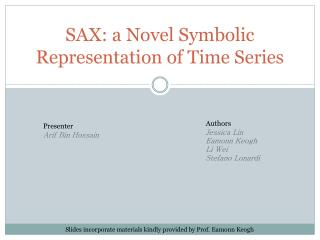DownloadDownload PresentationSAX: a Novel Symbolic Representation of Time Series

# SAX: a Novel Symbolic Representation of Time Series

Télécharger la présentation## SAX: a Novel Symbolic Representation of Time Series

- - - - - - - - - - - - - - - - - - - - - - - - - - - E N D - - - - - - - - - - - - - - - - - - - - - - - - - - -
##### Presentation Transcript

1. SAX: a Novel Symbolic Representation of Time Series Authors Jessica Lin Eamonn Keogh Li Wei Stefano Lonardi Presenter Arif Bin Hossain Slides incorporate materials kindly provided by Prof. Eamonn Keogh

2. Time Series •  A time series is a sequence of data points, measured typically at successive times spaced at uniform time intervals. [Wiki] • Example: • Economic, Sales, Stock market forecasting • EEG, ECG, BCI analysis 30 20 10 0 2000 4000 6000 8000 0

3. Problems Join: Given two data collections, link items occurring in each Annotation: obtain additional information from given data Query by content: Given a large data collection, find the k most similar objects to an object of interest. Clustering: Given a unlabeled dataset, arrange them into groups by their mutual similarity

4. Problems (Cont.) Classification: Given a labeled training set, classify future unlabeled examples Anomaly Detection: Given a large collection of objects, find the one that is most different to all the rest. Motif Finding: Given a large collection of objects, find the pair that is most similar.

5. Data Mining Constraints For example, suppose you have one gig of main memory and want to do K-means clustering… Clustering ¼ gig of data, 100 sec Clustering ½ gig of data, 200 sec Clustering 1 gig of data, 400 sec Clustering 1.1 gigs of data, few hours Bradley, M. Fayyad, & Reina: Scaling Clustering Algorithms to Large Databases. KDD 1998: 9-15

6. Generic Data Mining • Create an approximation of the data, which will fit in main memory, yet retains the essential features of interest • Approximately solve the problem at hand in main memory • Make (hopefully very few) accesses to the original data on disk to confirm the solution

7. Some Common Approximation

8. Why Symbolic Representation? • Reduce dimension • Numerosity reduction • Hashing • Suffix Trees • Markov Models • Stealing ideas from text processing/ bioinformatics community

9. Symbolic Aggregate ApproXimation(SAX) • Lower bounding of Euclidean distance • Lower bounding of the DTW distance • Dimensionality Reduction • Numerosity Reduction baabccbc

10. SAX Allows a time series of arbitrary length n to be reduced to a string of arbitrary length w (w<<n) Notations

11. How to obtain SAX? • Step 1: Reduce dimension by PAA • Time series C of length n can be represented in a w-dimensional space by a vector Ć = ć1,…ćw • The ith element is calculated by • Reduce dimension from 20 to 5. The 2nd element will be

12. How to obtain SAX? Data is divided into w equal sized frames. Mean value of the data falling within a frame is calculated Vector of these values becomes the PAA C C 0 20 40 60 80 100 120

13. c c c b b b a a - - 0 0 40 60 80 100 120 20 How to obtain SAX? • Step 2: Discretization • Normalize Ć to have a Gaussian distribution • Determine breakpoints that will produce a equal-sized areas under Gaussian curve. Words: 8 Alphabet: 3 baabccbc

14. Distance Measure • Given 2 time series Q and C • Euclidean distance • Distance after transforming the subsequence to PAA

15. Distance Measure • Define MINDIST after transforming to symbolic representation • MINDIST lower bounds the true distance between the original time series

16. Numerosity Reduction • Subsequences are extracted by a sliding window • Sequences are mostly repetitive subsequence • Sliding window finds aabbcc • If the next sequence is also aabbcc, just store the position • This optimization depends on the data, but typically yields a reduction factor of 2 or 3 • Space shuttle telemetry with subsequence length 32

17. Experimental Validation • Clustering • Hierarchical • Partitional • Classification • Nearest neighbor • Decision tree • Motif discovery

18. Hierarchical Clustering Sample dataset consists 3 decreasing trend, 3 upward shift and 3 normal classes

19. Partitional Clustering (k-means) Assign each point to one of k clusters whose center is nearest Each iteration tries to minimize the sum of squared intra-clustered error

20. Nearest Neighbor Classification SAX beats Euclidean distance due to the smoothing effect of dimensional reduction

21. Decision Tree Classification Since decision trees are expensive to use with high dimensional dataset, Regression Tree [Geurts.2001] is a better approach for data mining on time series

22. Motif Discovery • Implemented the random projection algorithm of Tompa and Buhler [ICMB2001] • Hashing subsequenced into buckets using a random subset of their features as a key

23. New Version: iSAX Use binary numbers for labeling the words Different alphabet size(cardinality)within a word Comparison of words with different cardinalities

24. Thank you Questions?